# 方差分析

CBT EMDR
s1 s6
s2 s7
s3 s8
s4 s9
s5 s10

s1
s2
s3
s4
s5
s6
s7
s8
s9
s10

CBT s1
s2
s3
s4
s5
EMDR s6
s7
s8
s9
s10

## 2 单因素方差分析

### 2.1 推导过程

$Y_{ij} = a_i + e_{ij}, j = 1,...,n_i, i = 1,...,k\qquad(2.1)$
$a_i$表示水平$i$的理论平均值，称为水平$i$的效应。在小麦例子中，$a_i$就是品种$i$的平均亩产量，$e_{ij}$就是随机误差。并且我们假定：
$E(e_{ij})=0, 0

$H_0:a_1=a_2=\cdots=a_k \qquad (2.3)$

$SS= \sum_{i=1}^{k}\sum_{j=1}^{n_i}\left ( Y_{ij}-\bar{Y} \right )^2, \qquad \bar{Y}=\sum_{i=1}^{k}\sum_{j=1}^{n_i}Y_{ij}/n \qquad (2.4)$
$SS$愈大，表示$Y_{ij}$之间的差异越大。

$\bar{Y_i}=(Y_{i1}+Y_{i2}+\cdots+Y_{in})/n_i,\quad i=1, 2,\cdots,n \qquad (2.5)$
$\bar{Y_i}$可以视为对$a_i$的估计。把上述平方和做累加得：
$SS_e=\sum_{i=1}^{k}\sum_{j=1}^{n_i}\left ( Y_{ij}-\bar{Y_i} \right )^2 \qquad (2.6)$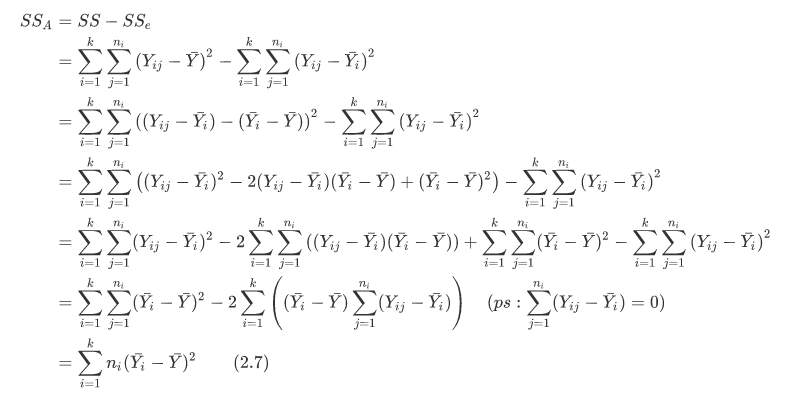$MS_A = SS_A/(k-1), \quad MS_e = SS_e/(n-k) \qquad (2.8)$
$H_0$成立时，有：
$MS_A / MS_e \sim F_{k-1, n-k} \qquad (2.9)$

$当MS_A / MS_e \leqslant F_{k-1, n-k}(\alpha)时，接受H_0，不然就拒绝H_0 \qquad (2.10)$
$MS_A$$MS_e$分别被称为因素$A$和随机误差的平均平方和。被除数$k-1$$n-k$，分别称为这两个平方和的自由度$MS_e$的自由度为什么是$n-k$呢？因为平方和$\sum_{j=1}^{n_i}\left ( Y_{ij}-\bar{Y_i} \right )^2$的自由度为$n_i-1$，故对$i$求和，$SS_e$的自由度就是$n-k$。那么，$MS_A$的自由度为什么是$k-1$呢？因为一共有$k$个平均值$a_1,\cdots,a_k$$k-1$个，故自由度为$k-1$，两者自由度之和为$n-1$，恰好是总平方和的自由度。

2-1 单因素方差分析的方差分析表

$A$ $SS_A$ $k-1$ $MS_A$ $MS_A / MS_e$ *, **, 或无

### 2.2 代码实例

#### 单因素方差分析的R语言实现

> library(multcomp)
> attach(cholesterol)
>
> # 统计各组样本大小
> table(trt)
trt
1time 2times 4times  drugD  drugE
10     10     10     10     10
>
> # 各组均值
> aggregate(response, by=list(trt), FUN=mean)
Group.1        x
1   1time  5.78197
2  2times  9.22497
3  4times 12.37478
4   drugD 15.36117
5   drugE 20.94752
>
> # 各组标准差
> aggregate(response, by=list(trt), FUN=sd)
Group.1        x
1   1time 2.878113
2  2times 3.483054
3  4times 2.923119
4   drugD 3.454636
5   drugE 3.345003
>
> # 进行方差分析
> fit <- aov(response ~ trt)
> summary(fit)
Df Sum Sq Mean Sq F value   Pr(>F)
trt          4 1351.4   337.8   32.43 9.82e-13 ***
Residuals   45  468.8    10.4
---
Signif. codes:  0 ‘***’ 0.001 ‘**’ 0.01 ‘*’ 0.05 ‘.’ 0.1 ‘ ’ 1


#### 查看各水平对应的组均值的差异

gplots包中的plotmeans()可以用来绘制带有置信区间的组均值图形。如图9-1所示，图形展示了带有95%的置信区间的各疗法均值，可以清楚看到它们之间的差异。

library(gplots)
plotmeans(response ~ trt, xlab="Treatment", ylab="Response",
main="Mean Plot\nwith 95% CI")
detach(cholesterol)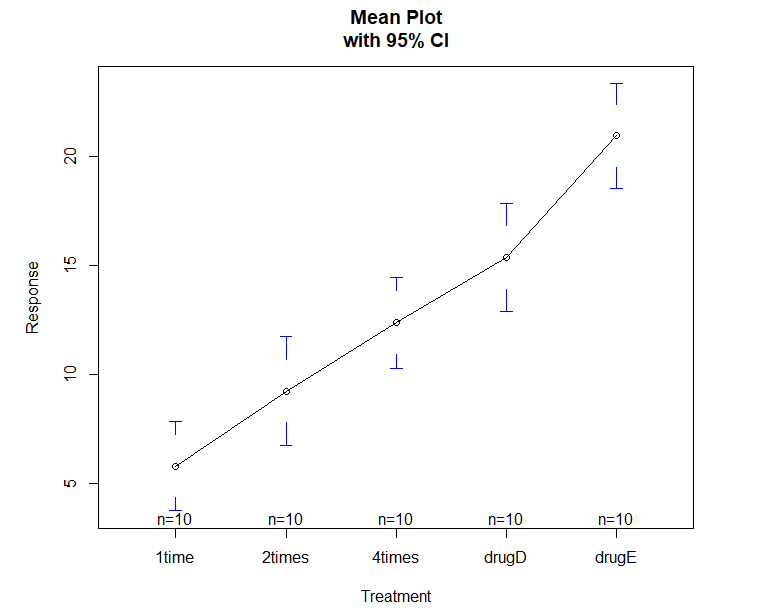2-1 五种降低胆固醇药物疗法的均值，含95%的置信区间

#### 多重比较

> TukeyHSD(fit)
Tukey multiple comparisons of means
95% family-wise confidence level

Fit: aov(formula = response ~ trt)

### 3.2 代码实例

> attach(ToothGrowth)
> table(supp, dose)
dose
supp 0.5  1  2
OJ  10 10 10
VC  10 10 10
>
> aggregate(len, by=list(supp, dose), FUN=mean)
Group.1 Group.2     x
1      OJ     0.5 13.23
2      VC     0.5  7.98
3      OJ     1.0 22.70
4      VC     1.0 16.77
5      OJ     2.0 26.06
6      VC     2.0 26.14
>
> aggregate(len, by=list(supp, dose), FUN=sd)
Group.1 Group.2        x
1      OJ     0.5 4.459709
2      VC     0.5 2.746634
3      OJ     1.0 3.910953
4      VC     1.0 2.515309
5      OJ     2.0 2.655058
6      VC     2.0 4.797731
>
> dose <- factor(dose)
#dose变量被转换为因子变量，这样aov()函数就会将它当做一个分组变量，而不是一个数值型协变量
> # condider interactive factor
> fit <- aov(len ~ supp*dose)
> summary(fit)
Df Sum Sq Mean Sq F value   Pr(>F)
supp         1  205.4   205.4  15.572 0.000231 ***
dose         2 2426.4  1213.2  92.000  < 2e-16 ***
supp:dose    2  108.3    54.2   4.107 0.021860 *
Residuals   54  712.1    13.2
---
Signif. codes:  0 ‘***’ 0.001 ‘**’ 0.01 ‘*’ 0.05 ‘.’ 0.1 ‘ ’ 1



# interactive effect
interaction.plot(dose, supp, len, type="b",
col=c("red","blue"), pch=c(16, 18),
main = "Interaction between Dose and Supplement Type")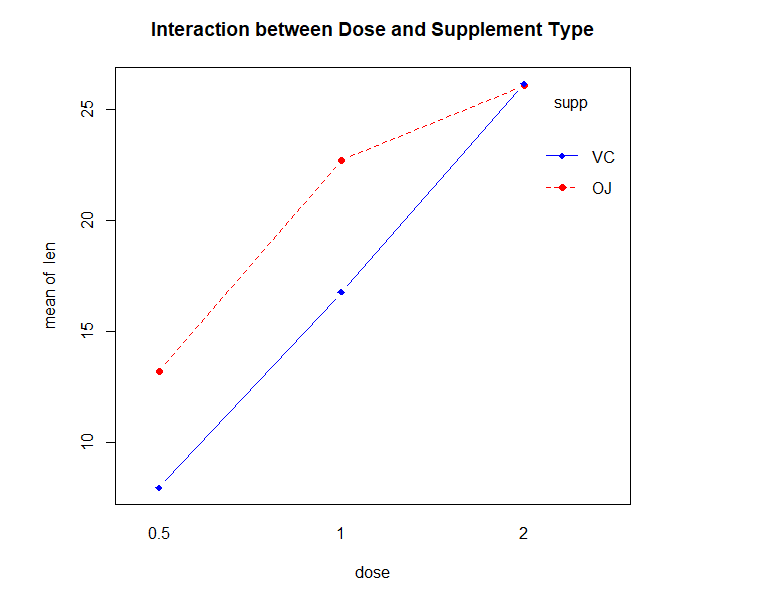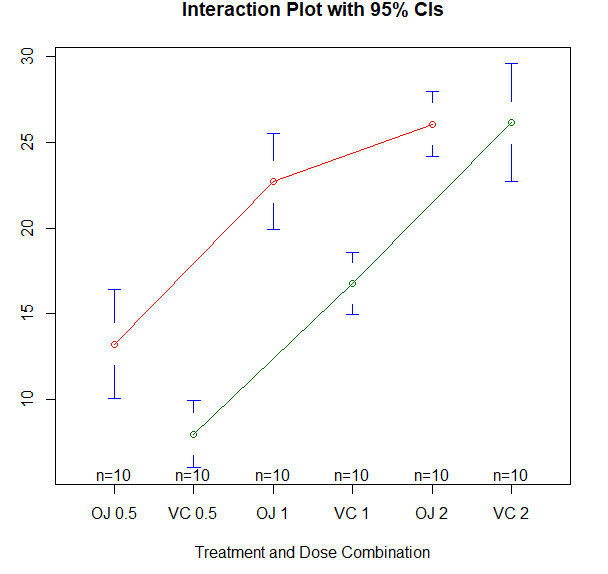library(HH)
interaction2wt(len~supp*dose)
>
> detach(ToothGrowth)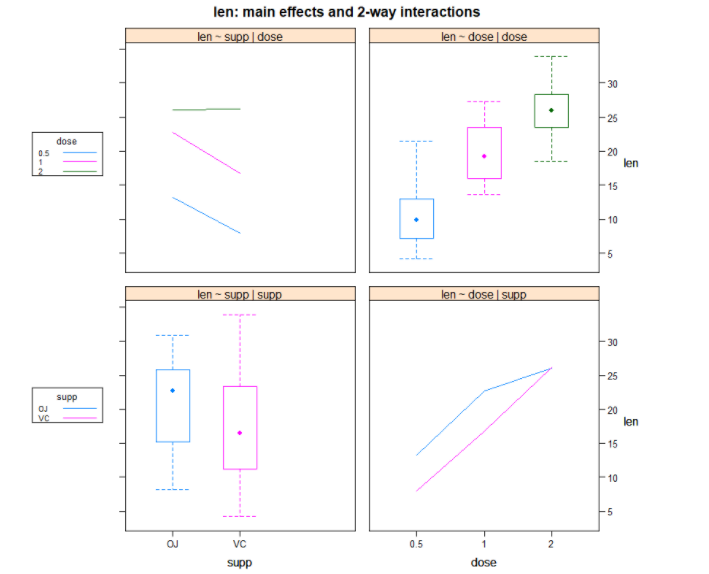#### 参考书目：

1. 陈希孺，概率论与数理统计
2. Robert I. Kabacoff, R in Action.

#### 史上最完整的人工智能学习路径，学习AI的请收藏好

07-08

03-014万+

#### 10.MATLAB方差分析05-212万+

#### 统计学习：ANOVA（方差分析）（1）

06-27148

#### 数据挖掘基础方法-概率统-4. 方差分析

11-011万+

#### Matlab方差分析

06-284373

#### 概率统计（四）方差分析

07-174万+

#### 方差分析以及如何用SPSS实现单因素、双因素方差分析

05-062085

#### 方差分析

04-12267

#### 方差分析原理及实例应用

04-02147

#### scipy.stats单因素方差分析

01-0953

#### C/C++学习指南全套教程

09-11

07-0938万+

#### Python可以这样学（第一季：Python内功修炼）

02-23©️2020 CSDN 皮肤主题: 数字20 设计师: CSDN官方博客点击重新获取扫码支付1.余额是钱包充值的虚拟货币，按照1:1的比例进行支付金额的抵扣。
2.余额无法直接购买下载，可以购买VIP、C币套餐、付费专栏及课程。余额充值